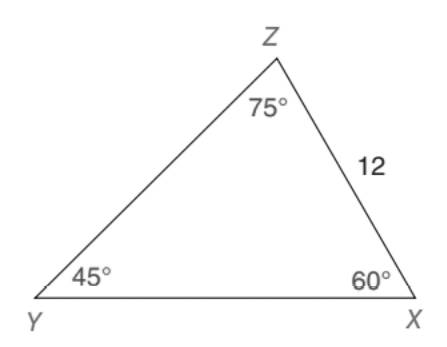Chapter 5.5, Problem 21EElementary Geometry For College St...

7th Edition
Alexander + 2 others
ISBN: 9781337614085

Solutions

Chapter
SectionElementary Geometry For College St...

7th Edition
Alexander + 2 others
ISBN: 9781337614085
Textbook Problem

Given: ∆ X Y Z with anglesas shown in thedrawing Find: X Y (HINT: Compare this drawing to the one for Exercise 20.)To determine

To find:

XY in XYZ with angles as shown in the drawing.

Explanation

Approach:

For a right triangle for which the measure of the interior angles 45°, 45°, and 90°, if ‘a’ is the length of measure of one of the leg; opposite to the angle 45°, then the length of the other two sides is given by

Length of the other leg =a

Length of the hypotenuse =a2

In general

Length of the legs are equal.

Length of the hypotenuse =2× (Length of one of the legs)

Calculation:

Given,

In XYZ,

mX=60°

mY=45°, and

mZ=75°.

Also, ZX=12 units.

Now, draw a perpendicular (ZW) from the vertex Z to the base XY of the XYZ, which divides the angle 75° at the vertex Z into two parts such that

mYZW=45° and mXZW=30°

Thus, XYZ is divided into two right triangles as YWZ and WXZ.

Here, YWZ is of 45°-45°-90° type of right and MPQ is of 30°-60°-90° type of right triangle.

Now, consider the triangle WXZ which has XZ is the hypotenuse and WZ is the leg opposite to the 60° angle and which is the longer leg and the leg WX is the shorter leg.

Thus, in WXZ

XZ= Hypotenuse =12 units.

WX= Shorter leg, and

WZ= Longer leg.

30°-60°-90° theorem.

In a right triangle whose angle measure 30°, 60°, and 90°, the hypotenuse has a length equal to twice the length of the shorter leg, and the longer leg is the product of 3 and the length of the shorter leg

Still sussing out bartleby?

Check out a sample textbook solution.

See a sample solution

The Solution to Your Study Problems

Bartleby provides explanations to thousands of textbook problems written by our experts, many with advanced degrees!

Get Started